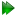Subsections

Frame order in rotational Brownian diffusion and NMR relaxation

Free ellipsoidal Brownian diffusion

In Perrin's equations for free ellipsoidal Brownian diffusion (Perrin, 1934,1936), the second degree frame order matrix elementsare an essential step of the derivation. From Perrin (1936), the solution for the ellipsoidal diffusion equation is+= e-(4, (12.66)=+2 + μie-6t +2 - μie-6t, (12.67)=-1 - μie-6t -1 + μie-6t, (12.68)

where

 μi =, (12.69)(12.70)(12.71)are the three diffusion rates, and cij are the direction cosines in the diffusion frame. According to Perrin (1936), because of the symmetry of the rotation the averages of the double-productswhere an index appears only once are zero and the second degree frame order matrix is represented by equation 12.15 on page. At time t = 0, the frame order matrix simplifies to

 Daeg(n)(0) = I1, (12.72)

and at time t = ∞ the matrix decays to

 Daeg(n)(∞) =I2. (12.73)

NMR relaxation

The free ellipsoid Brownian diffusion equations form the base theory for interpreting NMR relaxation data - the spheroidal and spherical diffusion equations are simply parametric restrictions of the full ellipsoid equations. As they are the definition of the frame order matrix, the frame order tensor can be seen as the modulator of all NMR relaxation processes.

The relax user manual (PDF), created 2020-08-26.